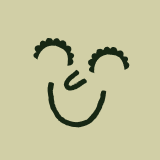teaching resource

# Mathematics Word Wall with Diagrams

• Updated:  30 Jan 2023

A set of 60 Mathematics-related vocabulary words with accompanying diagrams to explain key mathematical terms across three strands.

• Non-Editable:  PDF

• Pages:  22 Pages

• Years:  5 - 7

Tag #TeachStarter on Instagram for a chance to be featured!

teaching resource

# Mathematics Word Wall with Diagrams

• Updated:  30 Jan 2023

A set of 60 Mathematics-related vocabulary words with accompanying diagrams to explain key mathematical terms across three strands.

• Non-Editable:  PDF

• Pages:  22 Pages

• Years:  5 - 7

A set of 60 Mathematics-related vocabulary words with accompanying diagrams to explain key mathematical terms across three strands.

The three strands of mathematics included in this word wall are:

• Measurement and Geometry
• Number and Algebra
• Statistics and Probability.

Words include: circle, triangle, square, quadrilateral, pentagon, hexagon, heptagon, octagon, width, perimeter, area, length, volume, capacity, weight, sphere, cube, pyramid, grid, translation, reflection, rotation, angle, degree, factor, multiple, whole number, rounding, multiplication, division, addition, subtraction, remainder, fraction, decimal, number line, numerator, denominator, place value, less than, greater than, probability, data, observation, column graph, bar graph, table, line graph, pie chart.

Write a review to help other teachers and parents like yourself. If you'd like to request a change to this resource, or report an error, select the corresponding tab above.

••